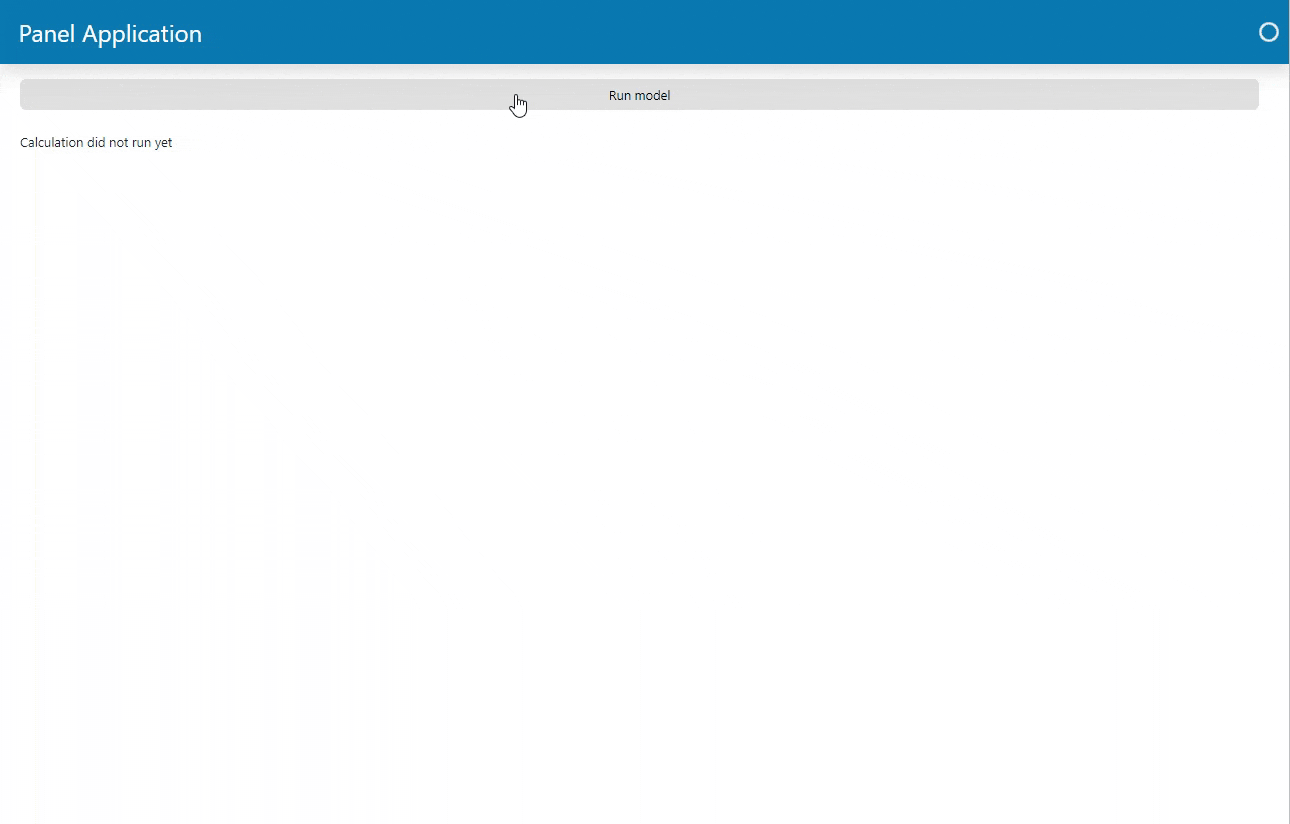# Add Interactivity with `pn.bind`#

Both Streamlit and Panel are reactive frameworks that react when you interact with your application. But they work very differently:

In Streamlit

• your script is run once when a user visits the page.

• your script is rerun top to bottom on user interactions.

In Panel

• your script is run once when a user visits the page.

• only specific, bound functions are rerun on user interactions.

With Panels interactivity architecture you will be able develop and maintain larger and more complex apps.

## Introduction#

Panels `pn.bind` provides the functionality to bind functions to widgets. We call the resulting functions bound functions.

You use `pn.bind` as follows

```# 1. Define your function(s)
def my_func(value):
return ...
slider = pn.widgets.IntSlider(...)
# 3. Bind your function to the widget(s)
my_bound_func = pn.bind(my_func, value=slider)
# 4. Layout and display your bound functions and widgets
pn.Column(slider, my_bound_func)
```

When you have bound and displayed your functions like above, they will automatically be rerun on user interactions.

## Migration Steps#

You should

• Move your business logic to functions. Business logic can be code to load data, transform data, run calculations, create plots, calculate the mass of the milky way, train models, do inference etc.

• Add interactivity by using `pn.bind` to bind your functions to widgets.

• Use generator functions (`yield`) if you want to update the UI multiple times during the functions execution.

• Indicate activity using the options described in the Show Activity Section.

## Examples#

### Basic Interactivity Example#

This example will show you how to migrate code that produces a single result and only updates the UI once the code execution has completed.

#### Streamlit Basic Interactivity Example#

```import matplotlib.pyplot as plt
import numpy as np
import streamlit as st

from matplotlib.figure import Figure

data = np.random.normal(1, 1, size=100)
fig = Figure(figsize=(8,4))
ax = fig.subplots()
bins = st.slider(value=20, min_value=10, max_value=30, step=1, label="Bins")
ax.hist(data, bins=bins)

st.pyplot(fig)
```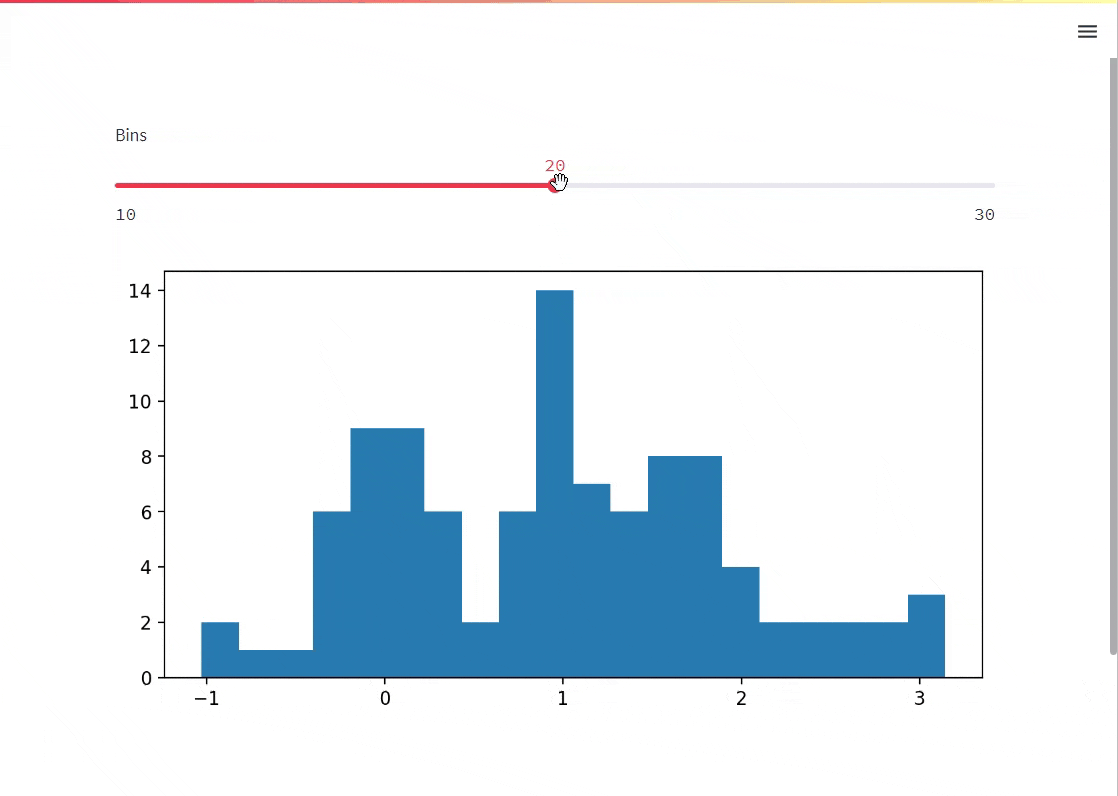The entire script is rerun top to bottom when you change the `bins` slider.

#### Panel Basic Interactivity Example#

```import panel as pn
import numpy as np

from matplotlib.figure import Figure

pn.extension(sizing_mode="stretch_width", template="bootstrap")

def plot(data, bins):
fig = Figure(figsize=(8,4))
ax = fig.subplots()
ax.hist(data, bins=bins)
return fig

data = np.random.normal(1, 1, size=100)
bins_input = pn.widgets.IntSlider(value=20, start=10, end=30, step=1, name="Bins")
bplot = pn.bind(plot, data=data, bins=bins_input)

pn.Column(bins, bplot).servable()
```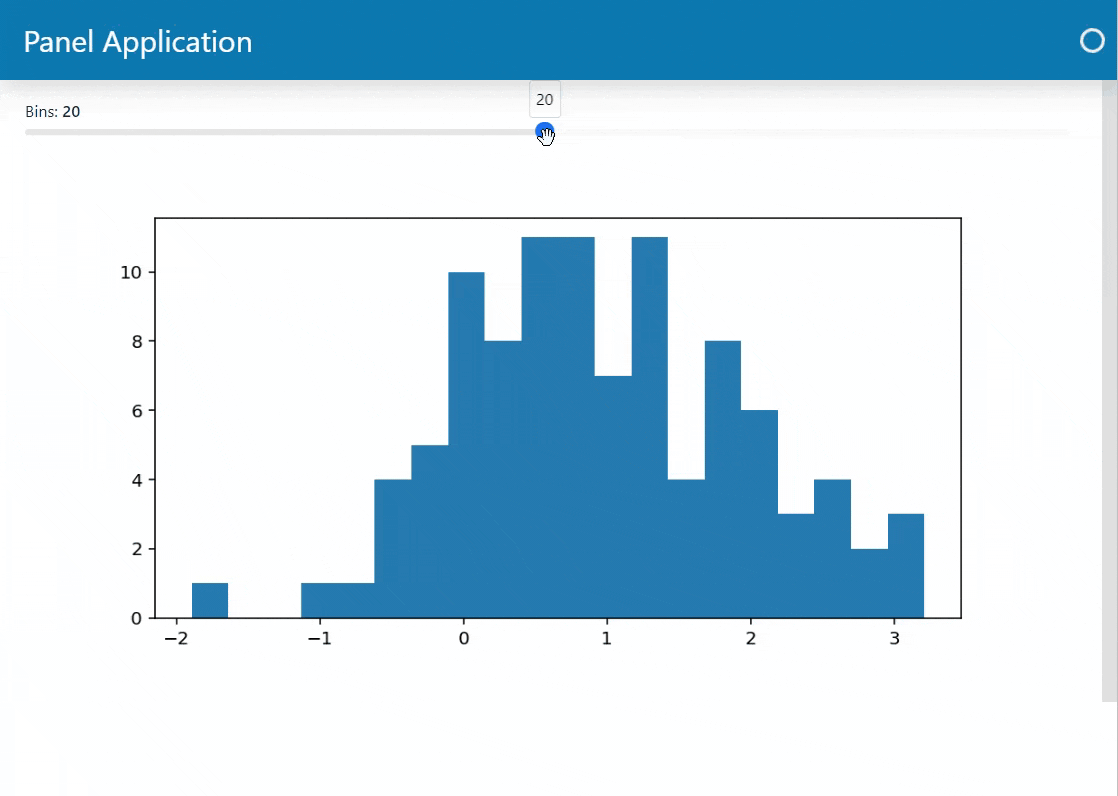With Panel only the `plot` function is rerun when you change the `bins` slider. This makes your Panel app update much quicker and more smoothly than the Streamlit app.

This example will show you how to migrate code that produces a single result and updates the UI multiple times during the code execution.

```import random
import time

import streamlit as st

def calculation_a():
time.sleep(1.5)
return random.randint(0, 100)

def calculation_b():
time.sleep(3.5)
return random.randint(-100, 0)

st.write("# Calculation Runner")
option = st.radio("Which calculation would you like to perform?", ("A", "B"))
st.write("You chose: ", option)
if st.button("Press to run calculation"):
time_start = time.perf_counter()
result = calculation_a() if option == "A" else calculation_b()
time_end = time.perf_counter()
st.write(f"""
Done!

Result: {result}

The function took {time_end - time_start:1.1f} seconds to complete
"""
)
else:
st.write(f"Calculation {option} did not run yet")
```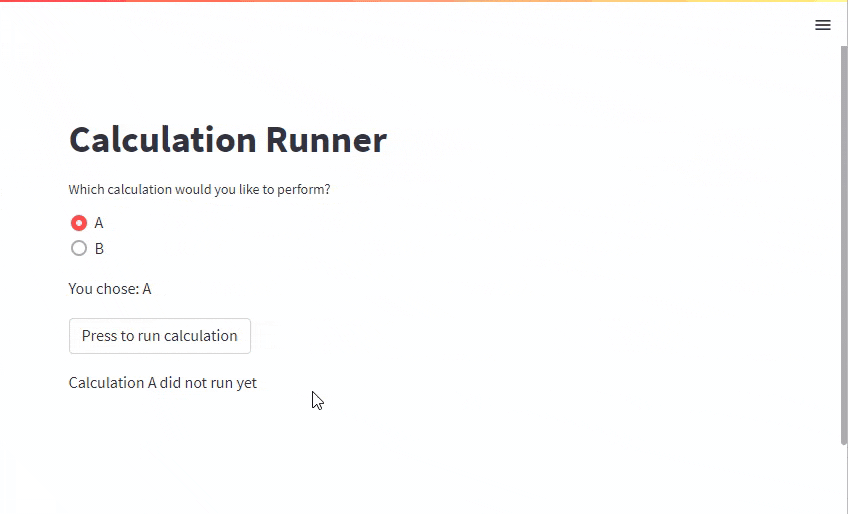With Panel you will use a generator function to update a component multiple times during code execution.

```
```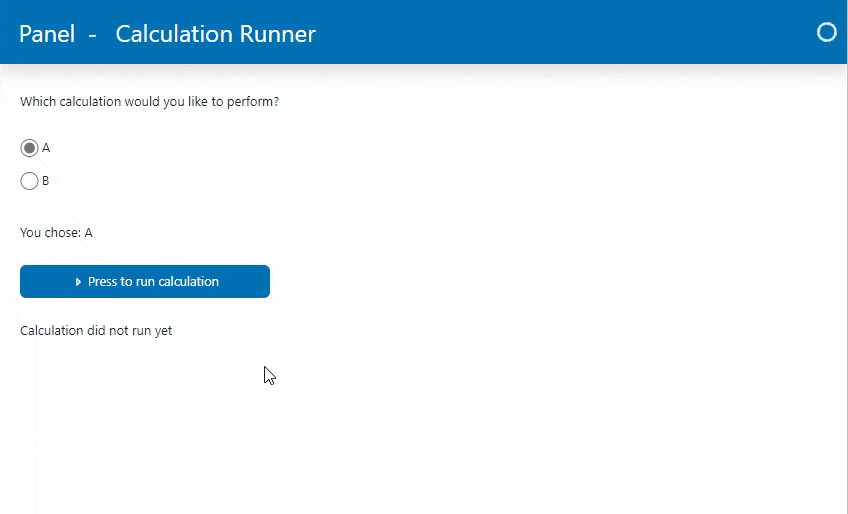You will notice that we use the `pn.indicators.LoadingSpinner` to indicate the activity.

#### Panel Multiple Updates Alternative Indicator Example#

An alternative to using an indicator would be to change the `.disabled` and `.loading` parameters of the `calculation_input` and `run_input`.

```import time
import random

import panel as pn

pn.extension(sizing_mode="stretch_width", template="bootstrap")
pn.state.template.param.update(site="Panel", title="Calculation Runner")

def notify_choice(calculation):
return f"You chose: {calculation}"

def calculation_a():
time.sleep(2)
return random.randint(0, 100)

def calculation_b():
time.sleep(1)
return random.randint(0, 100)

def run_calculation(running, calculation):
if not running:
yield "Calculation did not run yet"
return # This will break the execution

calc = calculation_a if calculation == "A" else calculation_b
)
time_start = time.perf_counter()
result = calc()
time_end = time.perf_counter()
yield f"""
Done!

Result: {result}

The function took {time_end - time_start:1.1f} seconds to complete
"""

run_input = pn.widgets.Button(
name="Press to run calculation",
icon="caret-right",
button_type="primary",
width=250,
)
pn.Column(
"Which calculation would you like to perform?",
calculation_input,
pn.bind(notify_choice, calculation_input),
run_input,
pn.bind(run_calculation, run_input, calculation_input),
).servable()
```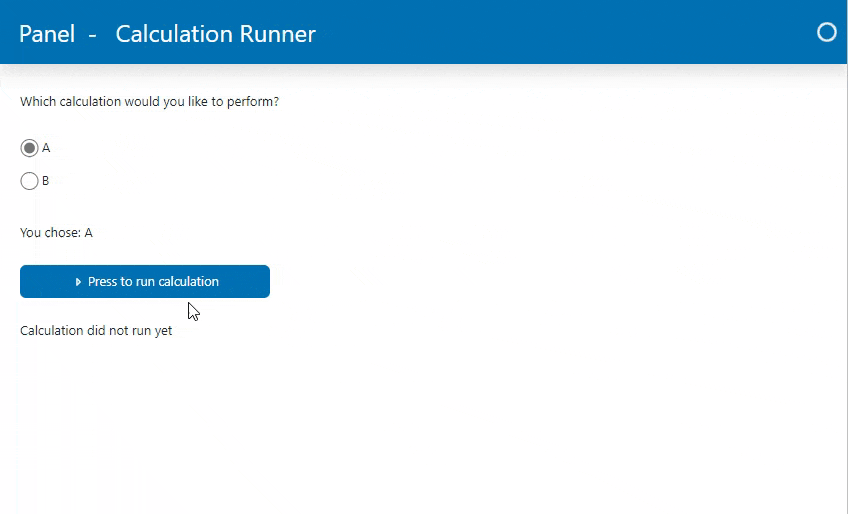### Multiple Results Example#

Sometimes you want to output multiple results individually as soon as they are ready.

This is for example the case for Large (AI) Language Models that generates one token after the other.

#### Streamlit Multiple Results Example#

```import random
import time

import streamlit as st

run = st.button("Run model")

def model():
time.sleep(1)
return random.randint(0, 100)

if not run:
st.write("The model has not run yet")
else:
with st.spinner("Running..."):
for i in range(0,10):
result = model()
st.write(f"Result {i}: {result}")
```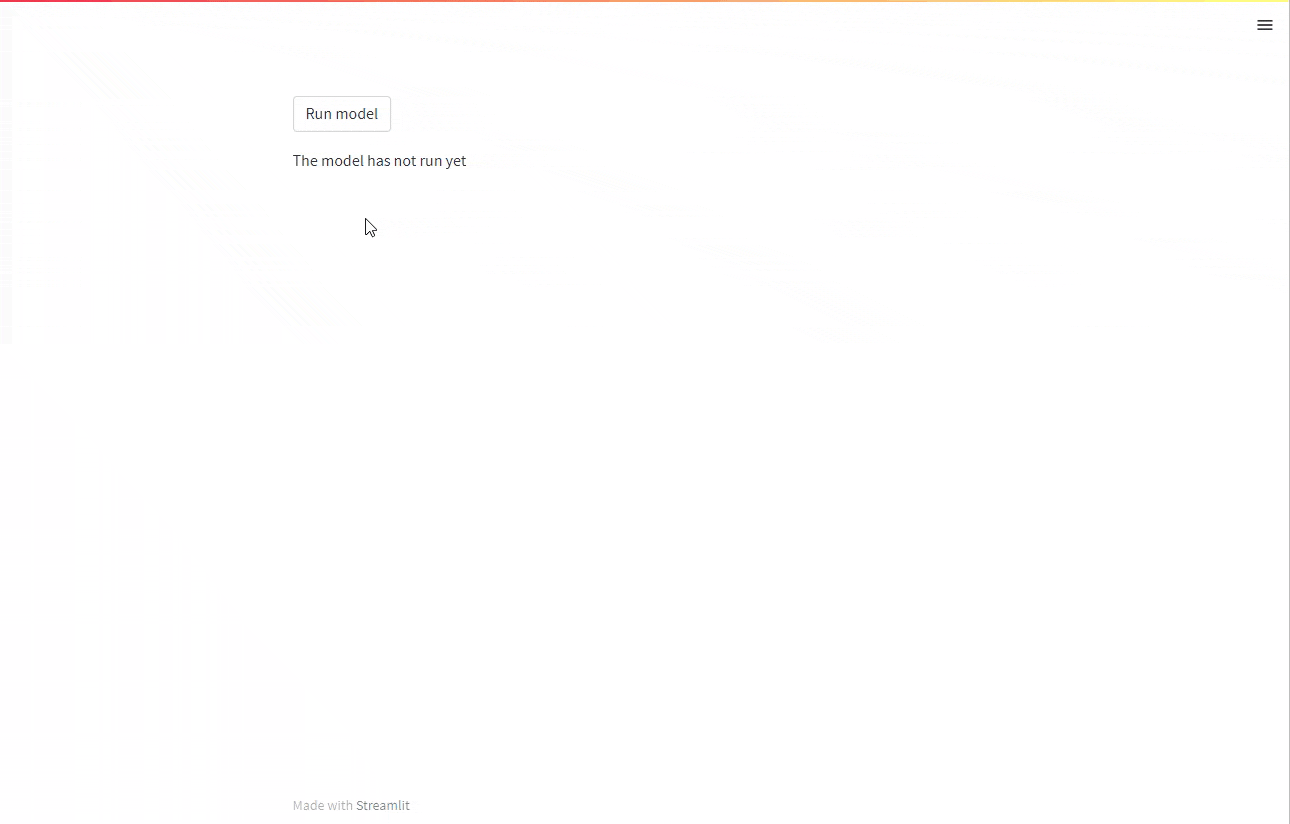#### Panel Multiple Results Example#

With Panel you will use a generator function to display multiple results from code execution as soon as they are ready.

```import random
import time
import panel as pn

pn.extension(sizing_mode="stretch_width")

def model():
time.sleep(1)
return random.randint(0, 100)

def results(running):
if not running:
return "The model has not run yet"

for i in range(0, 10):
result = model()
yield f"Result {i}: {result}"

run_input = pn.widgets.Button(name="Run model")
output = pn.bind(results, run_input)

pn.Column(
run_input,